### The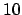-adic Numbers

It's a familiar fact that every real number can be written in the form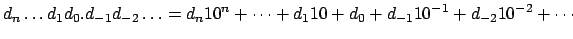where each digit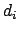is between 0 and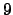, and the sequence can continue indefinitely to the right.

The-adic numbers also have decimal expansions, but everything is backward! To get a feeling for why this might be the case, we consider Euler's nonsensical series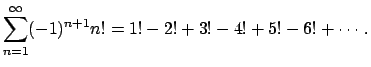One can prove (see Exercise 55) that this series converges in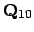to some element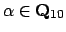.

What is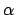? How can we write it down? First note that for all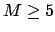, the terms of the sum are divisible by, so the difference betweenand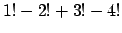is divisible by. Thus we can computemoduloby computingmodulo. Likewise, we can computemodulo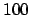by compute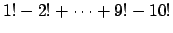, etc. We obtain the following table: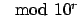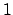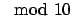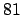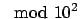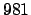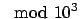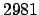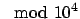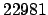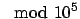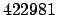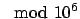Continuing we see that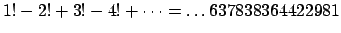in $Q_10$ !

Here's another example. Reducing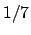modulo larger and larger powers ofwe see that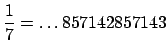in $Q_10$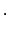Here's another example, but with a decimal point.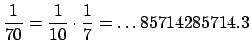We have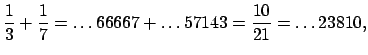which illustrates that addition with carrying works as usual.

William Stein 2004-05-06